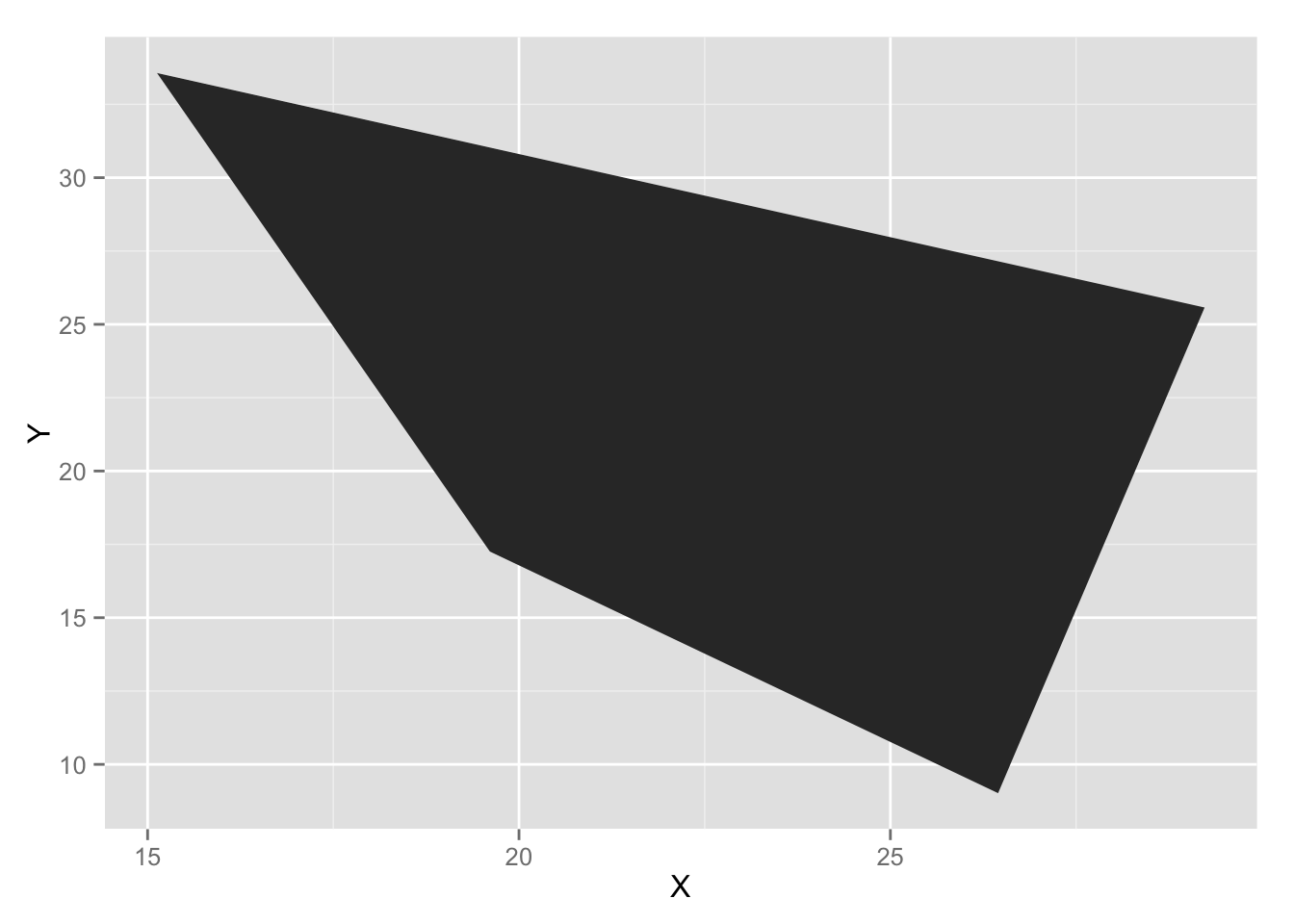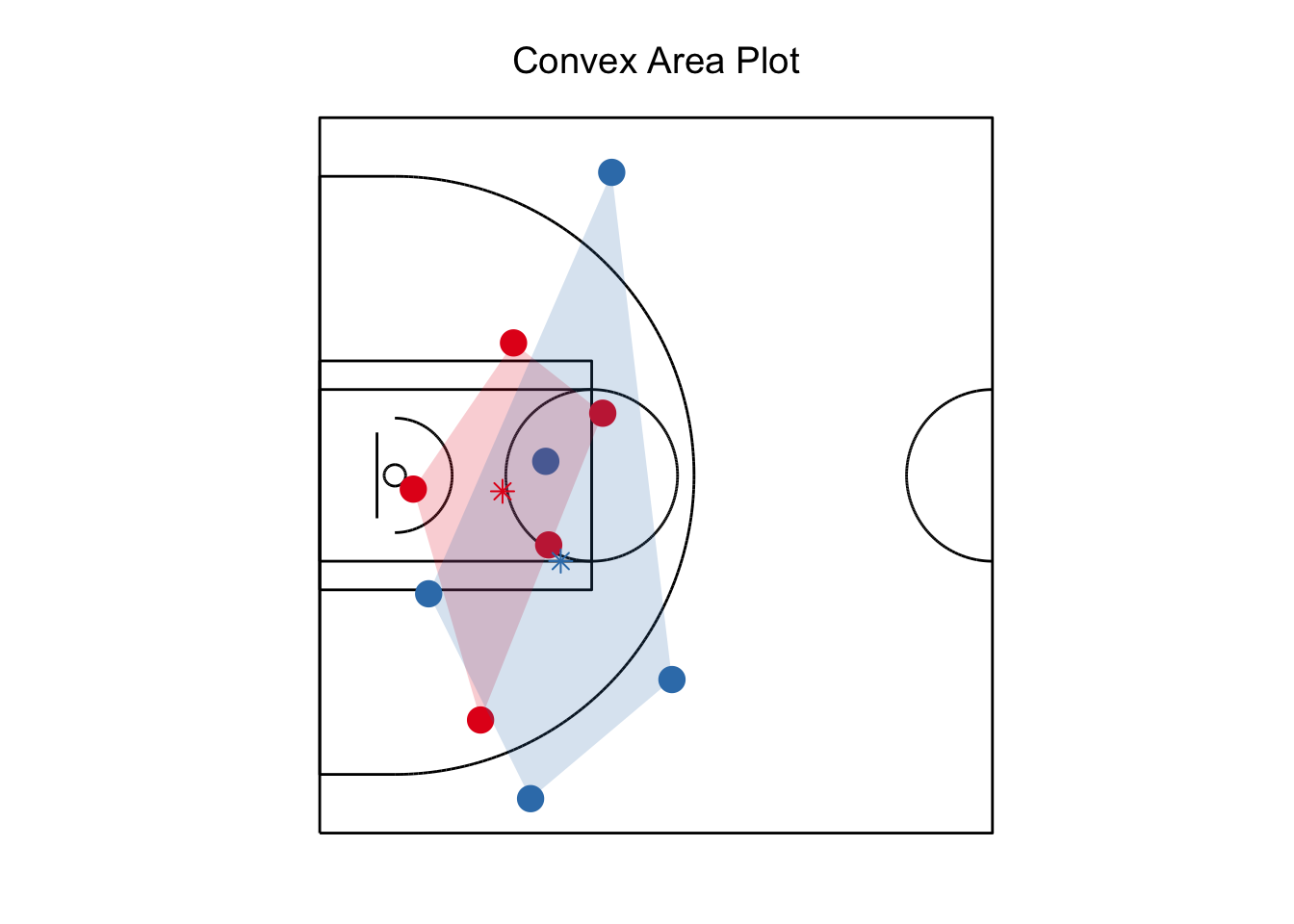This page shows how to measure the spacing distance using the concept of a convex hull measurement. Stephen Shea and Chris Baker explain this in their article. Take the players’ positions and create a convex hull around them. The area of the defensive polygon is termed Convex Hull Area of the Defense (CHAD) and the area of the offense is called the Convex Hull Area of the Offense (CHAO). Shea and Baker argue and show with limited data that the lineups that typically stretched the defense (CHAO much greater than CHAD) were very successful and efficient.

In this markdown, I want to show how to calculate these metrics using the SportsVU data. As a starting point, it is necessary to use my previous notebooks to grab the data and merge the play by play.

library(RCurl)
## Loading required package: bitops
library(jsonlite)
library(dplyr)
##
## Attaching package: 'dplyr'
##
## The following objects are masked from 'package:stats':
##
##     filter, lag
##
## The following objects are masked from 'package:base':
##
##     intersect, setdiff, setequal, union
library(sp)
library(ggplot2)
source("_functions.R")
source("_function_halfcourt.R")

### Grab the data for one event

Extract all data for event ID 303. Please refer to my other posts for how this data is downloaded and merged.

all.movements <- sportvu_convert_json("data/0021500431.json")
## Warning in FUN(X[[i]], ...): NAs introduced by coercion
gameid = "0021500431"
pbp <- get_pbp(gameid)
pbp <- pbp[-1,]
colnames(pbp) <- c('event.id')
#Trying to limit the fields to join to keep the overall size manageable
pbp <- pbp %>% select (event.id,EVENTMSGTYPE,EVENTMSGACTIONTYPE,SCORE)
pbp$event.id <- as.numeric(levels(pbp$event.id))[pbp$event.id] all.movements <- merge(x = all.movements, y = pbp, by = "event.id", all.x = TRUE) id303 <- all.movements[which(all.movements$event.id == 303),]

### Capture the players’ positions

The next step is capturing the player’s positions so the area can be calculated. For this example, I calculated it when the ball crossed the 28’ foot line (the top of the 3 point arc). The first step is finding the exact time the ball crossed the the line:

#Capture the first time they get to 28'
balltime <- id303 %>% group_by(event.id) %>% filter(lastname=="ball")  %>%
summarise(clock28 = max(game_clock[x_loc<28])) %>% print(event.id,clock28)
## Source: local data frame [1 x 2]
##
##   event.id clock28
##      (dbl)   (dbl)
## 1      303   373.4
#Find the positions of the players for each team at time 373.4 for event 303
dfall <- id303 %>% filter(game_clock == balltime$clock28) %>% filter(lastname!="ball") %>% select (team_id,x_loc,y_loc) colnames(dfall) <- c('ID','X','Y') head(dfall) ## ID X Y ## 1 1610612759 21.68490 10.82923 ## 2 1610612759 28.83188 6.48913 ## 3 1610612759 14.90278 45.55191 ## 4 1610612750 21.74513 29.49702 ## 5 1610612750 29.23077 25.57358 ## 6 1610612759 39.10015 25.94459 ### Calculate the Convex Hull R includes a number of geometry functions, including how to calculate the convex hull. For this example, lets calculate the convex hull for the defensive team. df_hull2 <- dfall %>% filter(ID == min(ID)) %>% select(X,Y) c.hull2 <- chull(df_hull2) #Calculates convex hull# c.hull3 <- c(c.hull2, c.hull2) #You need five points to draw four line segments, so we add the first set of points at the end df2 <- as.data.frame(cbind(1,df_hull2[c.hull3 ,]$X,df_hull2[c.hull3 ,]$Y)) colnames(df2) <- c('ID','X','Y') df2 # The points of the convex hull ## ID X Y ## 1 1 26.45010 9.01887 ## 2 1 19.60761 17.25700 ## 3 1 15.12674 33.56918 ## 4 1 29.23077 25.57358 ## 5 1 26.45010 9.01887 ggplot(df2, aes(x=X, y=Y)) + geom_polygon()### Get the area of the convex hull To use the convex hull feature, its important to be able to calculate its area and centroid.  chull.coords <- df_hull2[c.hull3 ,] chull.poly <- Polygon(chull.coords, hole=F) #From the package sp chull.area <- chull.poly@area chull.area ##  165.2116 ### Get the centroid of the convex hull The centroid is useful if you are trying to measure the defender’s average distance to the average position of the defense. Stephen and Chris refer to that as the DDA (for Defender’s Distance from Average). dfcentroid <- c(mean(df_hull2[c.hull2 ,]$X),mean(df_hull2[c.hull2 ,]$Y)) dfcentroid  ##  22.60381 21.35466 ### Plot this on a basketball court The area is easier to see on a court. To create this visualization, it is first necessary to create a background image of the basketball court and then overlay the players and convex hull plot. To do this, I created a number of functions that are on my github. I also slightly changed the time and did this 10 seconds later to highlight the difference in area each team controlled. ##These functions assume you have all the movement data in a data frame called total #Convert data into suitable format total <-id303 total$x_loc_r <- total$x_loc total$y_loc_r <- total$y_loc #Get data for building graphic dplayer <- player_position(303,361.11) #Gets positions of players dchull <- chull_plot(303,361.11) #Gets area of convex hull dcentroid <- chull_plot_centroid(303,361.11) #Gets centroid of convex hull #Plot graphic halfcourt() + ##Add players geom_point(data=dplayer,aes(x=X,y=Y,group=ID),color=dense_rank(dplayer$ID),size=5) + scale_colour_brewer() +
geom_polygon(data=dchull,aes(x=X,y=Y,group=ID),fill=dense_rank(dchull$ID),alpha = 0.2) + scale_fill_brewer() + ##Add Centroids scale_shape_identity() + geom_point(data=dcentroid,aes(x=X,y=Y,group=dcentroid$ID),color=(dcentroid\$ID),size=3,shape=8)### Build on this code

I used the above functions to calculate the differences in area by team for the game between San Antonio and Minnesota on Dec. 23rd.

I am still refining my code for calculating an entire game, but my first set of results found an average area of:
On makes: SAS: 356 versus MIN: 303
On misses: SAS: 326 versus MIN: 280

This is not surprising given that San Antonio won this game by a large margin.

### Credits

Thanks again to Steve and Chris for the writing about using convex hulls for analyzing basketball.

For more of my explorations on the NBA data you can see my NBA Github repo. You can find more information about me, Rajiv Shah or my other projects or find me on Twitter.